# NCERT Solutions for Class 10 Maths Exercise 3.4## myCBSEguide App

CBSE, NCERT, JEE Main, NEET-UG, NDA, Exam Papers, Question Bank, NCERT Solutions, Exemplars, Revision Notes, Free Videos, MCQ Tests & more.

NCERT solutions for Maths Pair of Linear Equations in Two Variables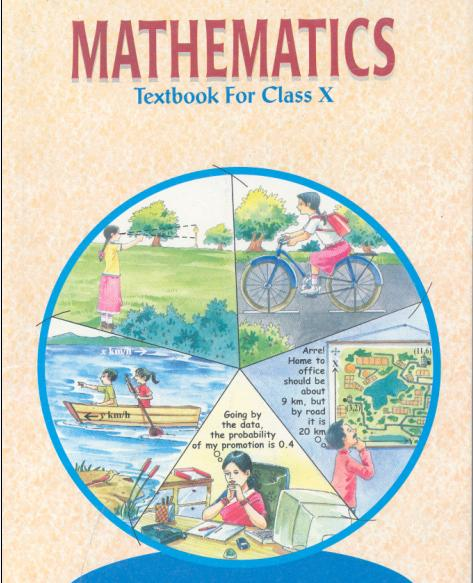## NCERT Solutions for Class 10 Maths Pair of Linear Equations in Two Variables

1. Solve the following pair of linear equations by the elimination method and the substitution method:

(i) x + y = 5, 2x – 3y = 4

(ii) 3x + 4y = 10, 2x – 2y = 2

(iii) 3x − 5y – 4 = 0, 9x = 2y + 7

(iv)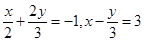Ans. (i) x + y = 5 … (1)

2x – 3y = 4 … (2)

Elimination method:

Multiplying equation (1) by 2, we get equation (3)

2x + 2y = 10 … (3)

2x − 3y = 4 … (2)

Subtracting equation (2) from (3), we get

5y = 6⇒ y =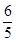Putting value of y in (1), we get

x += 5

x = 5 −=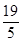Therefore, x =and y =###### Substitution method:

x + y = 5 … (1)

2x − 3y = 4 … (2)

From equation (1), we get,

x = 5 − y

Putting this in equation (2), we get

2 (5 − y) − 3y = 4

⇒ 10 − 2y − 3y = 4

⇒ 5y = 6 ⇒ y =Putting value of y in (1), we get

x = 5 −=Therefore, x =and y =(ii) 3x + 4y = 10… (1)

2x – 2y = 2… (2)

Elimination method:

Multiplying equation (2) by 2, we get (3)

4x − 4y = 4 … (3)

3x + 4y = 10 … (1)

Adding (3) and (1), we get

7x = 14⇒ x = 2

Putting value of x in (1), we get

3 (2) + 4y = 10

⇒ 4y = 10 – 6 = 4

y = 1

Therefore, x = 2 and y = 1

Substitution method:

3x + 4y = 10… (1)

2x − 2y = 2… (2)

From equation (2), we get

2x = 2 + 2y

x = 1 + y … (3)

Putting this in equation (1), we get

3 (1 + y) + 4y = 10

⇒ 3 + 3y + 4y = 10

⇒ 7y = 7⇒ y = 1

Putting value of y in (3), we get x = 1 + 1 = 2

Therefore, x = 2 and y = 1

(iii) 3x − 5y – 4 = 0 … (1)

9x = 2y + 7… (2)

Elimination method:

Multiplying (1) by 3, we get (3)

9x − 15y – 12 = 0… (3)

9x − 2y – 7 = 0… (2)

Subtracting (2) from (3), we get

−13y – 5 = 0

⇒ −13y = 5

y =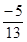Putting value of y in (1), we get

3x – 5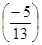− 4 = 0

⇒ 3x = 4 −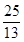=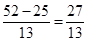x =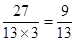Therefore, x =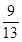and y =NCERT Solutions for Class 10 Maths Exercise 3.4

###### Substitution Method:

3x − 5y – 4 = 0 … (1)

9x = 2y + 7… (2)

From equation (1), we can say that

3x = 4 + 5yx =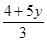Putting this in equation (2), we get

9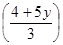− 2y = 7

⇒ 12 + 15y − 2y = 7

⇒ 13y = −5 ⇒ y =Putting value of y in (1), we get

3x – 5= 4

⇒ 3x = 4 −=x =Therefore, x =and y =(iv)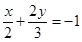… (1)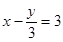… (2)

NCERT Solutions for Class 10 Maths Exercise 3.4

###### Elimination method:

Multiplying equation (2) by 2, we get (3)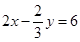… (3)… (1)

Adding (3) and (1), we get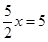x = 2

Putting value of x in (2), we get

2 −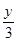= 3

y = −3

Therefore, x = 2 and y = −3

NCERT Solutions for Class 10 Maths Exercise 3.4

###### Substitution method:… (1)… (2)

From equation (2), we can say that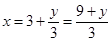Putting this in equation (1), we get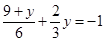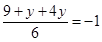⇒ 5y + 9 = −6

⇒ 5y = −15⇒ y = −3

Putting value of y in (1), we get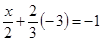x = 2

Therefore, x = 2 and y = −3

NCERT Solutions for Class 10 Maths Exercise 3.4

###### 2. Form the pair of linear equations in the following problems, and find their solutions (if they exist) by the elimination method:

(i) If we add 1 to the numerator and subtract 1 from the denominator, a fraction reduces to 1. It becomes ½ if we only add 1 to the denominator. What is the fraction?

(ii) Five years ago, Nuri was thrice as old as sonu. Ten years later, Nuri will be twice as old as sonu. How old are Nuri and Sonu?

(iii) The sum of the digits of a two–digit number is 9. Also, nine times this number is twice the number obtained by reversing the order of the digits. Find the number.

(iv) Meena went to a bank to withdraw Rs 2000. She asked the cashier togive her Rs 50 and Rs 100 notes only. Meena got 25 notes in all. Find how many notes of Rs 50 and Rs 100 she received.

(v) A lending library has a fixed charge for the first three days and an additional charge for each day thereafter. Saritha paid Rs 27 for a book kept for seven days, while Susy paid Rs 21 for the book she kept for five days. Find the fixed charge and the charge for each extra day.

Ans. (i) Let numerator =x and let denominator =y

According to given condition, we have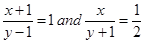x + 1 = y – 1 and 2x = y + 1

x y = −2 … (1) and 2x y = 1… (2)

So, we have equations (1) and (2), multiplying equation (1) by 2 we get (3)

2x − 2y = −4… (3)

2x y = 1… (2)

Subtracting equation (2) from (3), we get

y = −5⇒ y = 5

Putting value of y in (1), we get

x – 5 = −2⇒ x = −2 + 5 = 3

Therefore, fraction =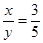NCERT Solutions for Class 10 Maths Exercise 3.4

(ii) Let present age of Nuri = x years and let present age of Sonu = y years

5 years ago, age of Nuri = (x – 5) years

5 years ago, age of Sonu = (y – 5) years

According to given condition, we have

(x − 5) = 3 (y − 5)

x – 5 = 3y – 15

x − 3y = −10… (1)

10 years later from present, age of Nuri = (x + 10) years

10 years later from present, age of Sonu = (y + 10) years

According to given condition, we have

(x + 10) = 2 (y + 10)

x + 10 = 2y + 20

x − 2y = 10 … (2)

Subtracting equation (1) from (2), we get

y = 10 − (−10) = 20 years

Putting value of y in (1), we get

x – 3 (20) = −10

x – 60 = −10

x = 50 years

Therefore, present age of Nuri = 50 years and present age of Sonu = 20 years

NCERT Solutions for Class 10 Maths Exercise 3.4

(iii) Let digit at ten’s place = x and Let digit at one’s place = y

According to given condition, we have

x + y = 9 … (1)

And 9 (10x + y) = 2 (10y + x)

⇒ 90x + 9y = 20y + 2x

⇒ 88x = 11y

⇒ 8x = y

⇒ 8x y = 0 … (2)

Adding (1) and (2), we get

9x = 9⇒ x = 1

Putting value of x in (1), we get

1 + y = 9

y = 9 – 1 = 8

Therefore, number = 10x + y = 10 (1) + 8 = 10 + 8 = 18

NCERT Solutions for Class 10 Maths Exercise 3.4

(iv) Let number of Rs 100 notes = x and let number of Rs 50 notes = y

According to given conditions, we have

x + y = 25 … (1)

and 100x + 50y = 2000

⇒ 2x + y = 40 … (2)

Subtracting (2) from (1), we get

x = −15⇒ x = 15

Putting value of x in (1), we get

15 + y = 25

y = 25 – 15 = 10

Therefore, number of Rs 100 notes = 15 and number of Rs 50 notes = 10

(v) Let fixed charge for 3 days = Rs x

Let additional charge for each day thereafter = Rs y

According to given condition, we have

x + 4y = 27 … (1)

x + 2y = 21 … (2)

Subtracting (2) from (1), we get

2y = 6⇒ y = 3

Putting value of y in (1), we get

x + 4 (3) = 27

x = 27 – 12 = 15

Therefore, fixed charge for 3 days = Rs 15 and additional charge for each day after 3 days = Rs 3

## NCERT Solutions for Class 10 Maths Exercise 3.4

NCERT Solutions Class 10 Maths PDF (Download) Free from myCBSEguide app and myCBSEguide website. Ncert solution class 10 Maths includes text book solutions from Mathematics Book. NCERT Solutions for CBSE Class 10 Maths have total 15 chapters. 10 Maths NCERT Solutions in PDF for free Download on our website. Ncert Maths class 10 solutions PDF and Maths ncert class 10 PDF solutions with latest modifications and as per the latest CBSE syllabus are only available in myCBSEguide.

## CBSE app for Class 10

To download NCERT Solutions for Class 10 Maths, Computer Science, Home Science,Hindi ,English, Social Science do check myCBSEguide app or website. myCBSEguide provides sample papers with solution, test papers for chapter-wise practice, NCERT solutions, NCERT Exemplar solutions, quick revision notes for ready reference, CBSE guess papers and CBSE important question papers. Sample Paper all are made available through the best app for CBSE students and myCBSEguide website.### 14 thoughts on “NCERT Solutions for Class 10 Maths Exercise 3.4”

1. Nice job Injamamul Hoque

2. Thanks for it .It’s so helpful

3. Nyc

4. Thank you for helping me.

5. Thanks for helping me

6. Plz give me the right answer of exercise 3.4. question number is 1.

7. some more clarity is an essential in process of solutions.Anyhow it will be very useful to every student….Thank you sir/madam

8. Thank you sir / mam but in que number 3 nuri aur sonu ki saaliyo ki maa ki chut kutti ki bachiya sala mc

9. Thanks for helping me

10. Thanks for helping me

11. Thank you for helping me